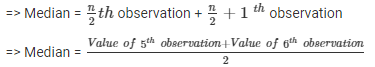# Test: Measures Of Central Tendency

## 10 Questions MCQ Test Mathematics (Maths) Class 9 | Test: Measures Of Central Tendency

Description
This mock test of Test: Measures Of Central Tendency for Class 9 helps you for every Class 9 entrance exam. This contains 10 Multiple Choice Questions for Class 9 Test: Measures Of Central Tendency (mcq) to study with solutions a complete question bank. The solved questions answers in this Test: Measures Of Central Tendency quiz give you a good mix of easy questions and tough questions. Class 9 students definitely take this Test: Measures Of Central Tendency exercise for a better result in the exam. You can find other Test: Measures Of Central Tendency extra questions, long questions & short questions for Class 9 on EduRev as well by searching above.
QUESTION: 1

### The mean of prime numbers between 20 and 30 is:

Solution:

The prime numbers between 20 and 30 is 23 , 29.
Mean of 23 and 29 = ( 23 + 29) / 2
= 52 / 2
= 26

QUESTION: 2

Solution:
QUESTION: 3

### In an examination, ten students scored the following marks: 60, 58, 90, 51, 47, 81, 70, 95, 87, 99. The range of this data is

Solution:

Difference between the maximum & minimum value of the observation is called as range.

So, 99-47=52

QUESTION: 4

If the number of observations in a data set is 25 and the mean of the data is 32. Then, the sum of all observations is ____________​

Solution: The no. of observation is 25
the mean is 32
the sum = 25 + 25 + 25... 32 times
i.e 32*25
= 800
QUESTION: 5

If the mean of 6, 4, 7, x and 10 is 8, the value of x is

Solution:

Mean = sum of all obs. /no. of obs.
8 = 6+4+7+x+10/5
40 = 27 +x
13 = x

QUESTION: 6

The maximum frequency 10 is for the observation 4. Hence the mode of the data is

Solution:
QUESTION: 7

Median of the data 5, 9, 8, 6, 3, 5, 7, 12, 15 is

Solution: Arranging the given terms in descending order 15 12 9 8 7 6 5 5 3
number of terms 9
median n+1/2=10/2
=5th term = 7
QUESTION: 8

Find median of following data: 83, 37, 70, 29, 45, 63, 41, 70, 34, 54

Solution:

Arranging the data in ascending order, we have:

29, 34, 37, 41, 45, 54, 63, 70, 70, 83

Here, the number of observations, n = 10 (Even).Hence, the median of the given data is 49.5.

QUESTION: 9

What will be the mean of x, x + 2, x + 4, x + 6, x + 8, where the value of x is 12?

Solution: In the given question we need to find the mean .The formula for mean is. sum of all the observations ÷ no. of obsevations. In the given question number of observation is 5. For finding the sum of all the observations we need to add all the observations. For this we add the constants and the variable seperately. Variable is x, so sum of all the variable is 5xand sum of all the constants (2+4+6+8) is 20. So the total is 5x+20. But the value of x is given which is 12.On substiting the value of x we get 5×12+20=80. Mean= 80÷5=16
QUESTION: 10

The median of observations 17, 18, 20, 22, x+2, x+4, 31, 35, 37 is found to be 24. If the observations have been arranged in ascending order, the value of x will be​

Solution: